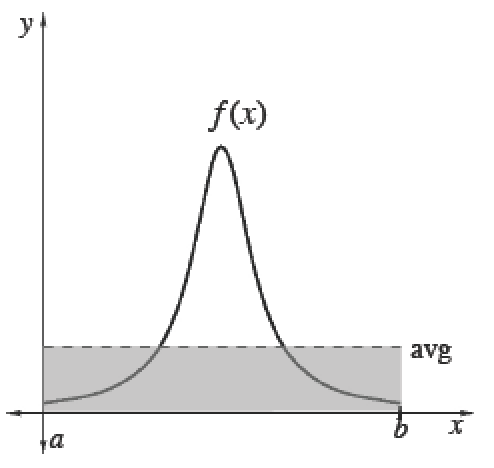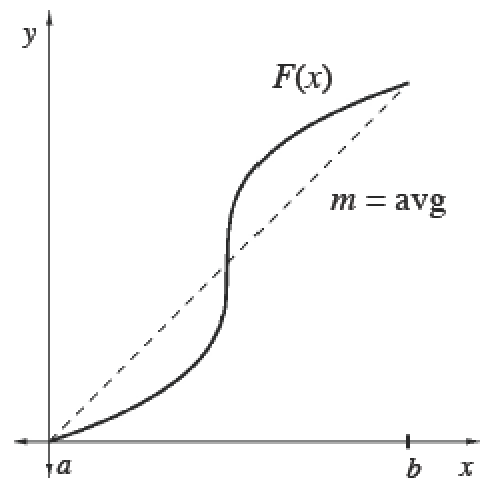### Home > CALC3RD > Chapter Ch7 > Lesson 7.3.6 > Problem7-169

7-169.During the semester, Arezo’s daily calculus grade at time, $t$ (in days), is given by $G(t) = 0.008t^2 + 22 \sin( \frac { t } { 5 } )+ 45$ . She will receive $50$ if she passes calculus, which requires an average grade of $60\%$ or better. If her semester grade (over $18$ weeks) is the average grade during that time period, should she celebrate?

$\$

$18 \times 7 = 126$ days

Find the average value of $G(t)$, given $G(t)$.

Average (Mean) Values

 To calculate the mean (average) value of a finite set of items, add up the values of items and divide by the number of items.Integrals help us add over a continuous interval. Therefore, for any continuous function $f$ :$\frac{\int_a^bf(x)dx}{b-a}=$ mean value of $f$ over $[a, b]$Since $\int _ { a } ^ { b } f ( x ) d x$, we can also calculate the average value of any function $f$ using its antiderivative $F$. Its average slope gives the average rate of change of $F$, which is the same as the average value of $f.$$\frac{\int_a^bf(x)dx}{b-a}=\frac { F ( b ) - F ( a ) } { b - a }=$ mean rate of change of $F ′$ over $[a, b]$